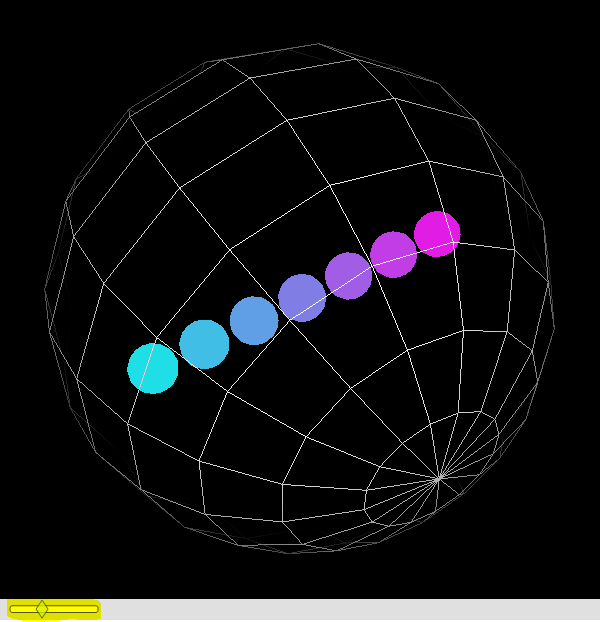Choose Language Hide Translation Bar
Highlighted

## How to hide scene3D objects?

I'm new to JSL programming and Opengl and was wondering about hiding objects. If I initially have multiple spheres in a scene, I want the user to be able to use a slider to specify the number of spheres in the scene, and update it accordingly. Is there a way to hide or delete objects?

1 ACCEPTED SOLUTION

Accepted Solutions
Highlighted

## Re: How to hide scene3D objects?

The display list does not have any logic of its own (for hiding/showing); you need to rebuild the displaylists to show what you want.

``````
sphere = Scene Display List();
sphere << sphere( .15, 15, 15 );

spheres = Scene Display List();
makespheres = Function( {n},
spheres << clear;
For( i = 1, i < n, i++,
spheres << color( i / n, 1 - i / n, .9 ); // .1,.1,.1 = almost black    .9,.9,.9 = almost white
spheres << pushmatrix;
spheres << translate( (i - (n / 2)) / 3, 0, 0 );
spheres << calllist( sphere );
spheres << popmatrix;
);
);

Scene = Scene Box( 600, 600 );
Scene << backgroundcolor( 0 ); // black=0, gray=1, white=2
Scene << showarcball( always );

nspheres = 14;
makespheres( nspheres );

New Window( "x",
Scene,
Slider Box(
1,
20,
nspheres,
makespheres( nspheres );
scene << update;
)
);
Scene << perspective( 45, .2, 20 );
Scene << Translate( 0.0, 0.0, -4.5 );
Scene << arcball( spheres, 1.5 );
Scene << update;``````Slider controls number of spheres

This uses several layers of called display lists. The littlest list, "sphere", just holds a single sphere, but might hold a more complicated object. The "spheres" list is the list that is rebuilt when the slider moves; keep it as simple as reasonable so the JSL loop doesn't have to work harder than needed when the slider moves. "Spheres", in turn, is called (via arcball) from the main display list. Another thing to notice: the called lists don't keep translations (etc) local to the called list. If a called list translates an object and doesn't undo that translation, the translation is still in effect when the called list is done. That's why the pushmatrix and popmatrix were used.

Craige
Highlighted

## Re: How to hide scene3D objects?

The display list does not have any logic of its own (for hiding/showing); you need to rebuild the displaylists to show what you want.

``````
sphere = Scene Display List();
sphere << sphere( .15, 15, 15 );

spheres = Scene Display List();
makespheres = Function( {n},
spheres << clear;
For( i = 1, i < n, i++,
spheres << color( i / n, 1 - i / n, .9 ); // .1,.1,.1 = almost black    .9,.9,.9 = almost white
spheres << pushmatrix;
spheres << translate( (i - (n / 2)) / 3, 0, 0 );
spheres << calllist( sphere );
spheres << popmatrix;
);
);

Scene = Scene Box( 600, 600 );
Scene << backgroundcolor( 0 ); // black=0, gray=1, white=2
Scene << showarcball( always );

nspheres = 14;
makespheres( nspheres );

New Window( "x",
Scene,
Slider Box(
1,
20,
nspheres,
makespheres( nspheres );
scene << update;
)
);
Scene << perspective( 45, .2, 20 );
Scene << Translate( 0.0, 0.0, -4.5 );
Scene << arcball( spheres, 1.5 );
Scene << update;``````Slider controls number of spheres

This uses several layers of called display lists. The littlest list, "sphere", just holds a single sphere, but might hold a more complicated object. The "spheres" list is the list that is rebuilt when the slider moves; keep it as simple as reasonable so the JSL loop doesn't have to work harder than needed when the slider moves. "Spheres", in turn, is called (via arcball) from the main display list. Another thing to notice: the called lists don't keep translations (etc) local to the called list. If a called list translates an object and doesn't undo that translation, the translation is still in effect when the called list is done. That's why the pushmatrix and popmatrix were used.

Craige
Article Labels

There are no labels assigned to this post.